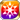# Pretty as a Picture

Type
Equipment
Rank
5★
Max level
40
Enhancer cost
2000
Enhancer EXP
20000
Vision Clear Reward
Source
Time Limited Summon
Release dates
• JP: 2018-06-14
• GL: 2019-06-27
AlchemistCodeDBThis is...me? Uzuma's voice was surprised, yet pleased. I knew it would be a good idea to have Scheherazade draw her portrait! They both seem to be having so much fun! We may have been summoned here to fight, but that doesn't mean we can't wear pretty outfits! (Letter from Eve to Moa)

### Pretty as a Picture

Raises HP by 40%/MATK by 40%/MDEF by 20%/Strong vs Gluttony by 20%/Strong vs Lust by 20%/Strong vs Envy by 20% for the Memento group "Desert Dwellers"

Target Buff (SelfSide) (CardLsSkill)
Stats
TypeMinMax
HP+40%+40%
MATK+40%+40%
MDEF+20%+20%
Strong vs <暴食>+20+20
Strong vs <嫉妬>+20+20
Strong vs <色欲>+20+20

## Stats

#### Simple stats

TypeLvl 1Lvl 30Lvl 40
MDEF+10+24+30

LevelMDEF
1+10
2+10
3+11
4+11
5+12
6+12
7+13
8+13
9+14
10+14
LevelMDEF
11+15
12+15
13+16
14+16
15+17
16+17
17+18
18+18
19+19
20+19
LevelMDEF
21+20
22+20
23+21
24+21
25+22
26+22
27+23
28+23
29+24
30+24
LevelMDEF
31+25
32+25
33+26
34+26
35+27
36+27
37+28
38+28
39+29
40+30

## Group skills

### Ace of the Desert Zone

#### Simple stats

TypeLvl 1Lvl 30Lvl 40
DEX+10+32+40

LevelDEX
1+10
2+10
3+11
4+12
5+13
6+13
7+14
8+15
9+16
10+16
LevelDEX
11+17
12+18
13+19
14+20
15+20
16+21
17+22
18+23
19+23
20+24
LevelDEX
21+25
22+26
23+26
24+27
25+28
26+29
27+30
28+30
29+31
30+32
LevelDEX
31+33
32+33
33+34
34+35
35+36
36+36
37+37
38+38
39+39
40+40

#### Limit Break

TypeLB1LB2LB3LB4LB5
DEX+5+9+14+19+24

### Wedding Vows

#### Simple stats

TypeLvl 1Lvl 30Lvl 40
PDEF+20+49+60
MDEF+10+24+30

LevelPDEFMDEF
1+20+10
2+21+10
3+22+11
4+23+11
5+24+12
6+25+12
7+26+13
8+27+13
9+28+14
10+29+14
LevelPDEFMDEF
11+30+15
12+31+15
13+32+16
14+33+16
15+34+17
16+35+17
17+36+18
18+37+18
19+38+19
20+39+19
LevelPDEFMDEF
21+40+20
22+41+20
23+42+21
24+43+21
25+44+22
26+45+22
27+46+23
28+47+23
29+48+24
30+49+24
LevelPDEFMDEF
31+50+25
32+51+25
33+52+26
34+53+26
35+54+27
36+55+27
37+56+28
38+57+28
39+58+29
40+60+30

#### Limit Break

TypeLB1LB2LB3LB4LB5
PDEF+7+14+21+28+36
MDEF+4+7+11+14+18

### Dream of the Future

#### Simple stats

TypeLvl 1Lvl 30Lvl 40
HP+60+164+200
Magic ATK Power+3+8+10

#### Detailed stats

LevelHPMagic ATK Power
1+60+3
2+63+3
3+67+3
4+70+3
5+74+3
6+77+3
7+81+4
8+85+4
9+88+4
10+92+4
LevelHPMagic ATK Power
11+95+4
12+99+4
13+103+5
14+106+5
15+110+5
16+113+5
17+117+5
18+121+6
19+124+6
20+128+6
LevelHPMagic ATK Power
21+131+6
22+135+6
23+138+6
24+142+7
25+146+7
26+149+7
27+153+7
28+156+7
29+160+8
30+164+8
LevelHPMagic ATK Power
31+167+8
32+171+8
33+174+8
34+178+8
35+182+9
36+185+9
37+189+9
38+192+9
39+196+9
40+200+10

#### Limit Break

TypeLB1LB2LB3LB4LB5
HP+24+48+72+96+120
Magic ATK Power+1+2+3+4+6

TypeLB5
Hit Rate+15

## Vision abilities

### Limit Reached Bonus

#### Ability base

• Slot:Support

Revealed after Limit Reached. Raises AGI after appearing on map.

#### Ability max

• Slot:Action

Raises AGI after appearing on map.